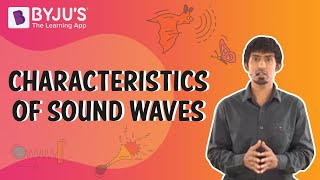# Beats Questions

The interference of sound waves generates the beat. When overlapping two waves of nearby frequencies create a new resultant wave, beats take place. The beat frequency is created when two sound waves with different frequencies come across each other, and here, the amplitude gets added and subtracted for a given time period.

1. What is the sound?

Sound in the form of energy responsible for soothing music and harsh noises around us.

2. What is meant by wave interference?

It is the phenomenon that takes place when two waves meet while traveling along with the same medium.

3. When A has a 700 Hz frequency and B has a 500 Hz frequency, what would be the resultant frequency if these two frequencies interfere in time.

1. 120 Hz
2. 20 Hz
3. 200 Hz
4. 1200 Hz

Explanation: The resultant frequency would be 700 Hz – 500 Hz = 200 Hz.

4. State true or false: The source of sound is vibration.

1. TRUE
2. FALSE

Explanation: Vibration is the cause of sound generation.

5. In music, the tone colour or tone quality is defined using which property?

1. Location
2. Frequency
3. Amplitude
4. Timbre

Explanation: Timbre is the perceived sound quality of a musical note, sound or tone.

6. The audible sound ranges between ______

1. 20 Hz to 20 kHz
2. 10 Hz to 12 kHz
3. More than 20 kHz
4. Less than 10 Hz

Answer: a) 20 Hz to 20 kHz

Explanation: The range of audible sound ranges from 20 Hz to 20 kHz.

7. The sound wave is below 20 Hz is classified as _______

1. Sonic
2. Supersonic
3. Infrasonic
4. None of the options

Explanation: Sound waves below 20 Hz frequency are known as infrasonic sound.

8. Choose the right answer: What is the range of ultrasonic?

1. Below 20 Hz
2. Above 20 Hz
3. Above 20 kHz
4. None of the options

Explanation: Sound above the frequency of 20 kHz is classified as ultrasonic.

9. List various properties of sound.

Properties of sound are:

• Timbre
• Location
• Duration
• Frequency
• Envelope
• Amplitude

10. How is wave interference classified?

Wave interference is classified as constructive interference and destructive interference.

## Characteristics of Sound Waves## Practice Questions

1. Define a wave?
2. How is sound classified?
3. Explain with an example the inaudible sound.
4. List the types of sound.
5. Explain the creation of beats.